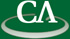## The Radius of Convergence of a Taylor Series

### Greg Fee, CECM, SFU

Wednesday October 3rd, 3:30pm in K9509.

```Abstract:

We will look at numerical methods for estimating the
radius of convergence of a truncated taylor series.  In particular, we
consider the series in x satisfying z-log(1+z) = x^2/2.  This series
begins with  x + x^2/3 + x^3/36 - x^4/270 + x^5/4320 + x^6/17010 + ...
which suggests that the radius of convergence is infinite but this
is not the case.

```